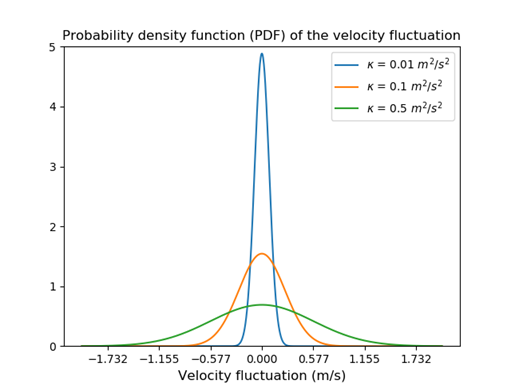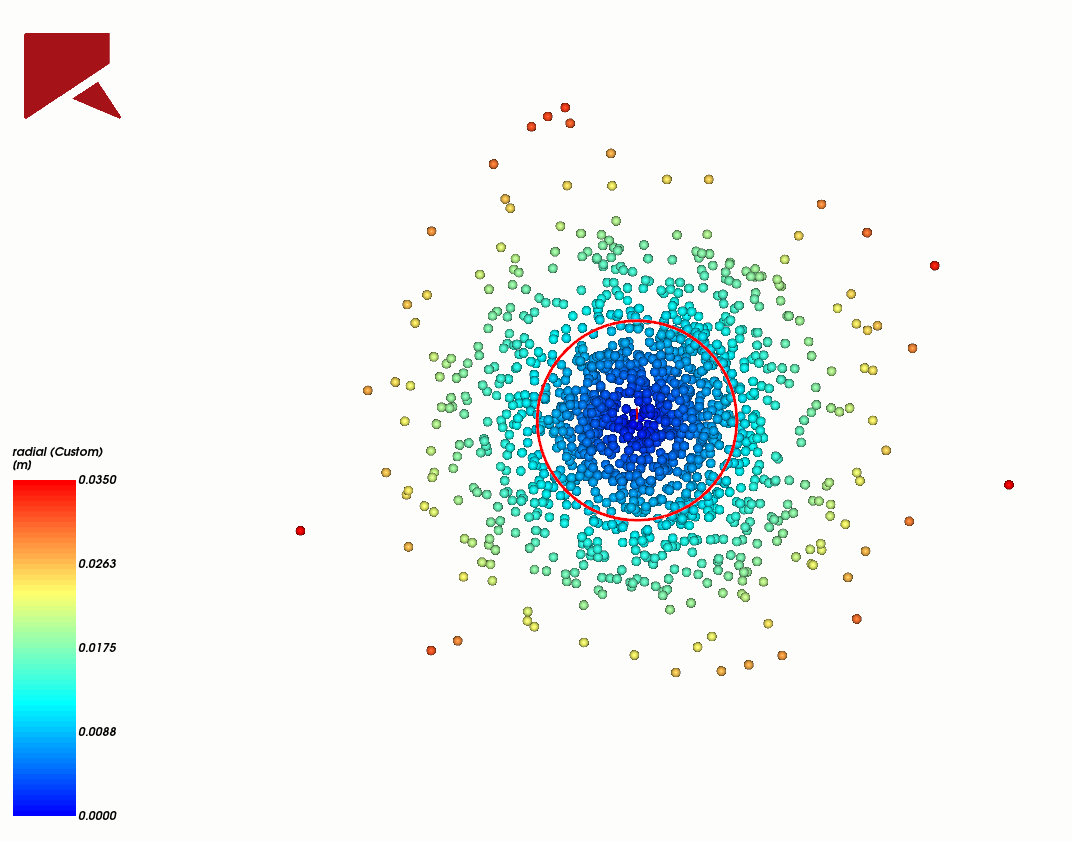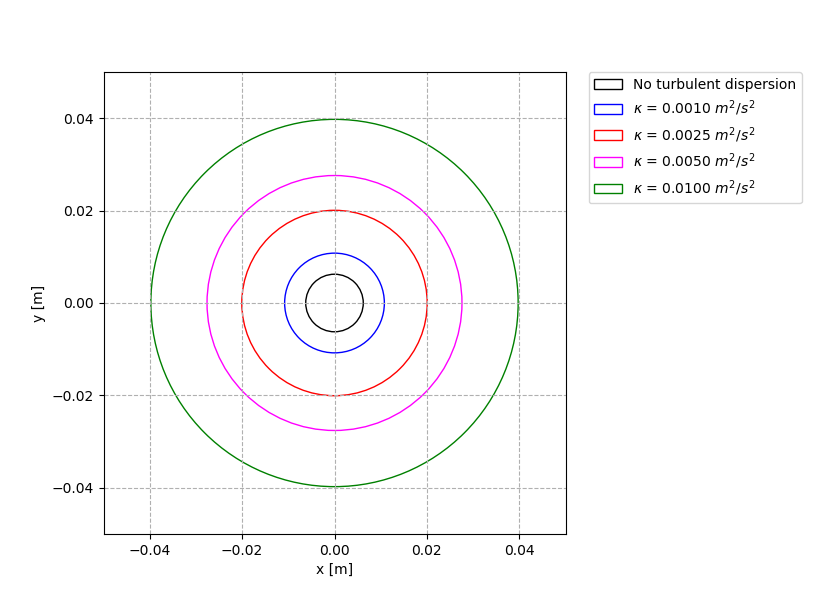## Turbulent dispersion model: discover how to account for the turbulence effect on particle trajectories

In many systems, it is important to account for the influence of the fluid phase when predicting particle trajectories. Due to the particle-fluid interactions, two additional terms appear in the equations that govern translational and rotational particle motion when compared with a DEM simulation that does not consider the presence of fluids: an additional net force accounting for the interaction with the fluid phase and an additional torque due to the fluid phase velocity gradient.

## Fluid forces acting on particles

When you’re solving a coupled DEM-CFD simulation in the current Rocky version, not only can the drag force be added to particles, but also the pressure gradient, lift, and virtual mass forces can be considered, depending on the flow conditions.

To compute drag and non-drag forces, not only are the local fluid properties (such as density and viscosity) needed, but also the local fluid velocity and the pressure are required.

The fluid velocity transferred to Rocky DEM from the CFD solver is obtained by solving the Reynolds-averaged Navier-Stokes (RANS) equations, and therefore is the time-averaged velocity at each fluid cell, at each fluid time step.

And here lies the issue.

## A little bit about turbulence

Turbulent flows are characterized by eddies that decay into smaller eddies and so forth, which then combine to form the famous turbulent energy cascade. These turbulent eddies are born and die constantly, which creates flow characteristics such as randomness and unsteadiness. Each vortex has its own velocity and lifetime, and the distribution probability of both depends on the turbulent characteristics of the fluid flow.

Figure 1 illustrates the problem we face: on the left, you can see a snapshot of a jet of water directed downward into the water. On the right, you see the results obtained in a CFD simulation using the RANS approach. In this case, although the time-average velocity is correctly computed, the randomness due to the turbulence is filtered out. This affects the trajectory of the particles, as this randomness is the main dispersion mechanism in dispersed flows (on the macroscopic level).Figure 1. (left) A jet of water directed downward into water with Reynolds number approximately 2300 (obtained in Van Dyke, M. and Van, D., An Album of Fluid Motion, 1982); (right) the same jet simulated in CFD using the RANS approach.

## How to incorporate the turbulent effect?

When using the turbulent dispersion model implemented in Rocky (also known as a discrete random walk [DRW] model, or “eddy lifetime” model), the interaction of a particle with a succession of discrete stylized fluid phase turbulent eddies is simulated.
Each eddy is characterized by:
• a Gaussian distributed random velocity fluctuation
• a time scale.
To incorporate the turbulent dispersion, a fluctuating velocity term, u’, is added to the average fluid velocity, u:

u = u + u’

The fluctuating velocity is computed according to the local turbulence field (κ and ε) and is kept constant over an interval of time given by the characteristic lifetime of the eddies.

Returning to Figure 1: The idea of the turbulent dispersion model is, in some sense, to mimic the effect of the unsteadiness and randomness of the velocity field shown in the left image, given the averaged velocity field displayed in the right image.

## Estimating the fluctuation velocity magnitude

For computing the fluctuation magnitude, the turbulence is assumed to be isotropic. The fluctuation magnitude velocity is estimated assuming it follows a normal distribution around zero with standard deviation given by the fluid turbulent kinetic energy, κ. The direction of the fluctuating velocity is assumed to be a random variable.
This way, the higher the local turbulent kinetic energy, the higher the fluctuation velocities that can be added to the average fluid velocity, as shown in Figure 2.Figure 2. The probability density function of the velocity fluctuation for different values of the turbulent kinetic energy (κ). This graph shows that the greater the turbulence intensity, the greater the fluctuation velocities that can be added to the average fluid velocity.

## Estimating the fluctuation period

The particle may remain within the same eddy during its whole lifetime, or the particle may cross the eddy before the eddy ceases to exist. Therefore, the particle-eddy interaction time is given as the smaller value between the particle crossing time and the eddy lifetime.

When this time period is reached, a new value for the fluctuation velocity is generated (mimicking the fact that a new turbulent eddy will be affecting the particle).

The eddy lifetime is estimated based on the dissipation length scale (which in turn is estimated based on the values of the turbulent kinetic energy and dissipation rate), whereas the particle crossing time is estimated based on the particle relaxation time.

## Illustrating the effect of the turbulent dispersion model in a simple case

The example below in Video 1 illustrates the effect of the turbulent dispersion model on the dispersion of very small particles. It shows a one-way coupled simulation in which 1-mm particles were injected through a 10-mm inlet section with 1 m/s in the z-direction. The water flows to the right with a constant velocity of 1 m/s and the turbulent kinetic energy was set to values in the range of 0.001 m2/s2 to 0.01 m2/s2.

It can be seen that, without the addition of the turbulent dispersion model, the particles move to the right with the same velocity, and no dispersion occurs. When the turbulent dispersion model is turned on, the particles still move to the right following the water flow, but the random fluctuations added to the water velocity cause the particles to move in other directions, causing the stream to open wide, which better matches the actual behavior.

In order to compare the influence of the turbulent kinetic energy on the particles, the radial position of the particles was monitored at a section located 1 m downwards the inlet region, as shown in Figure 3. The average radial position of the particles for different turbulent kinetic energies is plotted in Figure 4. As expected, the greater the turbulence intensity, the larger the velocity fluctuations can become. The higher the values of the velocity fluctuation components, the more the particles spread out.Figure 3. The radial position of the particles 1m downwards the inlet region.Figure 4. The average radial position of the particles 1 m downwards the inlet region for different turbulent kinetic values.

For more details on the turbulent dispersion model, such as how the fluctuation magnitude and period are estimated, refer to the Rocky CFD Coupling Technical Manual.

### Lucilla Almeida

CAE Specialist at ESSS, D.Sc.

Lucilla holds a BE (Chemical) undergraduate degree, an M.Sc. in Chemical Engineering and a Ph.D. in Nuclear engineering from the Federal University of Rio de Janeiro. She joined ESSS in 2008 and has spent 5 years focused on applying CFD to solve common engineering problems in the Oil and Gas industry, dealing with turbulent and multiphase flow simulations. Since 2013, she is an Application Engineer for Rocky DEM Business Unit, supporting users, working on consultancy projects and validating models implemented for the CFD-DEM coupling.